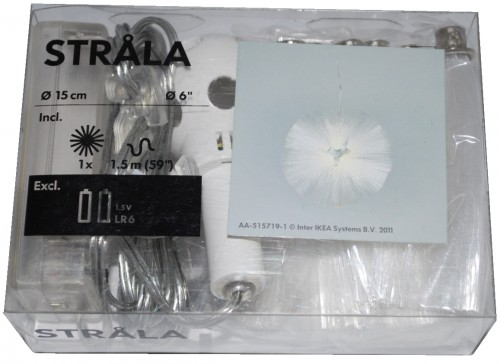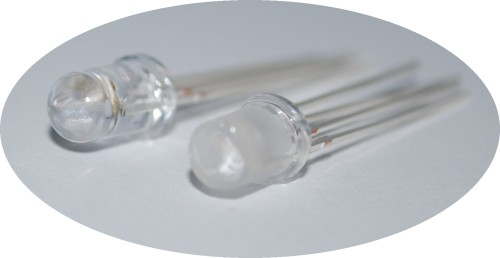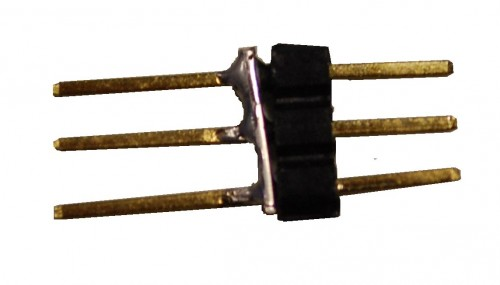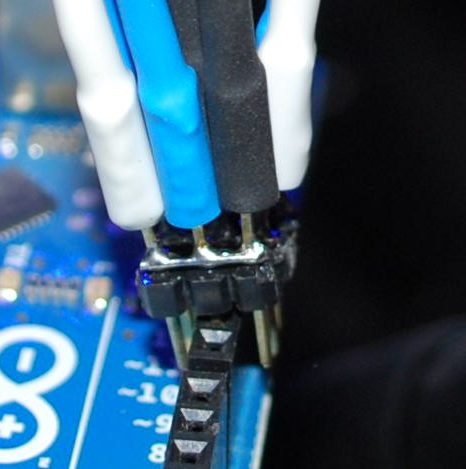# IKEA lamp mod – STRÅLA

By on November 9, 2011I love IKEA and her low cost products.

Last week I found this STRÅLA lamp.The cost is \$4.99, so I bought it.
Lighting the lamp I saw that it ‘s very pretty but also … too soft…So I thought to modify the LED and add a RGB led.
With simple modify you can obtain a dinamic lamp.
I used an Arduino to do a fast work, but of course it isn’t necessary.
You can use a small microcontroller like a PIC12F675, or a ATTiny.

And this it the result:

How to

Before all, you have to buy a STRÅLAOpen the packageAnd disassemble the original LEDAs you can see the LED shape isn’t normal, but there is a cone in the center to better spread the light.
So I modify the RGB led to obtain the same effects and I also smoothed the surface.Connect the wire to the led and reassemble all.

To connect the RGB led I used a strip wire and some pin.And now you can connect this pin to PWM ports of Arduino.The black, blue and white wire are the RGB pin of the LED.

The red one is the Anode.The sketch

```/* IKEA Strala mods

created 2011
by Boris Landoni

This example code is in the public domain.

http://www.open-electronics.org
http://www.futurashop.it
http://blog.elettronicain.it/
*/

#include <EEPROM.h>

int red = 9;    // RED LED connected to PWM pin 3
int green = 10;    // GREEN LED connected to PWM pin 5
int blue = 11;    // BLUE LED connected to PWM pin 6
int r=50; int g=100; int b=150;
int rup; int gup; int bup;
int fader=1;
int inc=10;
void setup()
{
// start the Ethernet connection and the server:
Serial.begin(9600);
Serial.println("Serial READY");
r = EEPROM.read(1);
g = EEPROM.read(2);
b = EEPROM.read(3);
inc = EEPROM.read(4);
fader = EEPROM.read(5);
rgb(r, g, b);
}

void loop()  {

if (fader==1){
funcfader();
}

}

void funcfader(){
Serial.println("fader");
if (rup==1){r+=1;}
else{r-=1;}
if (r>=255){rup=0;}
if (r<=0){rup=1;}

if (gup==1){g+=1;}
else{g-=1;}
if (g>=255){gup=0;}
if (g<=0){gup=1;}

if (bup==1){b+=1;}
else{b-=1;}
if (b>=255){bup=0;}
if (b<=0){bup=1;}

delay(inc*2);
rgb(r, g, b);
}

void rgb(int r, int g, int b)
{

Serial.print("RGB: ");
Serial.print(r);
Serial.print(" ");
Serial.print(g);
Serial.print(" ");
Serial.print(b);
if (r>255) r=255;
if (g>255) g=255;
if (b>255) b=255;
if (r<0) r=0;
if (g<0) g=0;
if (b<0) b=0;

analogWrite(red, r);
analogWrite(green, g);
analogWrite(blue, b);
}```

Good modding#### About Boris Landoni

Boris Landoni is the technical manager of Open-Electronics.org. Skilled in the GSM field, embraces the Open Source philosophy and its projects are available to the community.